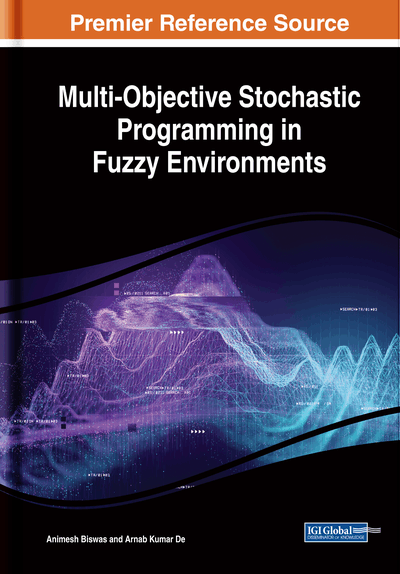# Fuzzy Multi-Objective Programming With Joint Probability Distribution

DOI: 10.4018/978-1-5225-8301-1.ch007
Available
\$33.75
List Price: \$37.50
10% Discount:-\$3.75
TOTAL SAVINGS: \$3.75

## Abstract

In this chapter, a fuzzy goal programming (FGP) model is employed for solving multi-objective linear programming (MOLP) problem under fuzzy stochastic uncertain environment in which the probabilistic constraints involves fuzzy random variables (FRVs) following joint probability distribution. In the preceding chapters, the authors explain about linear, fractional, quadratic programming models with multiple conflicting objectives under fuzzy stochastic environment. But the chance constraints in these chapters are considered independently. However, in practical situations, the decision makers (DMs) face various uncertainties where the chance constraints occur jointly. By considering the above fact, the authors presented a solution methodology for fuzzy stochastic MOLP (FSMOLP) with joint probabilistic constraint following some continuous probability distributions. Like the other chapters, chance constrained programming (CCP) methodology is adopted for handling probabilistic constraints. But the difference is that in the earlier chapters chance constraints are considered independently, whereas in this chapter all the chance constraints are taken jointly. Then the transformed problem involving possibilistic uncertainty is converted into a comparable deterministic problem by using the method of defuzzification of the fuzzy numbers (FNs). Objectives are now solved independently under the set of modified system constraints to obtain the best solution of each objective. Then the membership function for each objective is constructed, and finally, a fuzzy goal programming (FGP) model is developed for the achievement of the highest membership goals to the extent possible by minimizing group regrets in the decision-making context.
Chapter Preview
Top

## 7.1 Fuzzy Stochastic Programming Following Joint Probability Distribution

Authors in the preceding chapters already clarified the fact that due to the coexistence of imprecision and uncertainty, the DMs have to deal with real life decision-making problems within hybrid uncertain environments. It is necessary to combine both probability theory and possibility theory for a hybrid uncertain decision making problems. Through FRV probabilistic and possibilistic uncertainty can be described simultaneously. FRV is a mapping from a probability space to a collection of fuzzy variables, hence acts as an appropriate and effective tool to deal with hybrid uncertainty in an optimization framework. The concept of FRV was first introduced by Zadeh (1968) using probability measure. After Zadeh, the thought of FRV was advanced by Kwakernaak (1978, 1979) which was further developed by Puri and Ralescu (1986), Liu and Iwamura (1998), Liu and Liu (2003), Buckley and Eslami (2004, 2004a) as per the different necessities of measurability.

Fuzzy CCP (FCCP) methodology is applied to those CCP problems in which at least one parameter of the constraints or the objective function has FRVs. CCP with both fuzziness and randomness was examined by Gharraph and Mohammed (1994), Liu and Iwamura (1998) and Mohammed (2000) and others. An interval-parameter dynamic CCP approach for capacity planning under uncertainty was developed by Dai et al. (2012). The researchers employ the FCCP technique to change over hybrid decision making problem into a fuzzy programming problem. As traditional linear programming, Zimmermann (1978) first introduced fuzzy linear programming (FLP). In that approach, LP problems are considered with a fuzzy goal and fuzzy constraints. Zimmermann (1983) proposed an approach for determining suitable values for the aspiration level and admissible violation of the fuzzy goal, rather than leaving this decision to DMs. After the pioneering work on FLP by Zimmermann (1983), several kinds of FLP problems appeared in the literature and different methods have been introduced to take care of such problems. A few of the methods depend on the idea of the penalty method (Jamison and Lodwick, 2001), the satisfaction degree of the constraints (Liu, 2001), the statistical confidence interval (Chiang, 2001), and optimization method with multiple objectives (Zhang et al, 2003). Other kinds of methods are semi-infinite programming method (Leon and Vercher, 2004), the superiority and inferiority of FNs (Hop, 2007), and the degrees of feasibility (Jimenez et al, 2007). Since then FLP has developed in various ways with numerous fruitful applications. Among the others, the approach of Verdegay (1982) and Chanas (1983) which proposed a parametric programming method for solving FLP is one of the most often used technique. Several researchers (Delgado et al., 1989; Lai and Hwang, 1992; Lai and Hwang, 1992a) studied FLP problem with fuzzy goals, fuzzy constraints and fuzzy parameters in a concise manner. FLP with fuzzy variables and the application of ranking functions in FLP were discussed in the literature (Campus and Verdegay, 1989; Cadenas and Verdegay, 2000; Maleki, 2003) by several researchers in the past.

## Complete Chapter List

Search this Book:
Reset